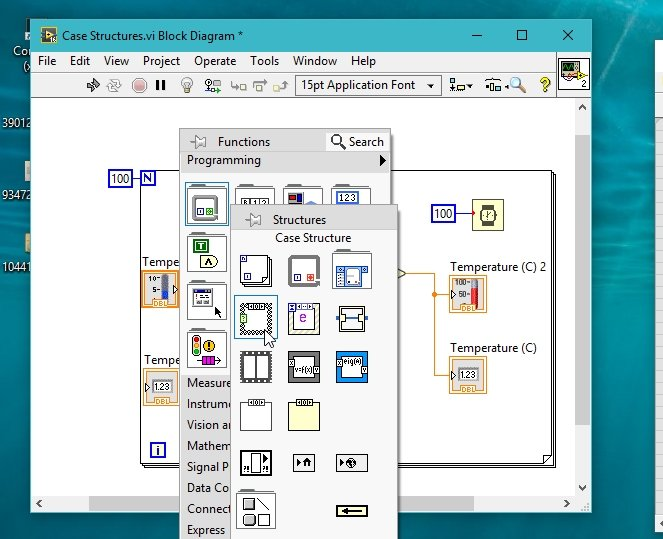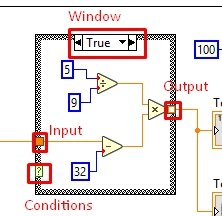# case structures examples in labview: tutorial 11

In this tutorial, you will learn the use of case structures. Case structures help us to selectively execute our code. A case structure will execute one part of the VI at one time, depending upon the condition of the case selector. We can execute one of many cases or one of two cases at a time, depending on if we use a numeric constant as a condition or a Boolean constant respectively. At the start I have given a brief but explanatory introduction of the case structures and compared their functionality with other programming environments. After that you are provided with a program that will use case structures and help you understand their uses in depth. At the end of the tutorial you are provided with an exercise to do it by yourself, and in the next tutorials I will assume that you have done those exercises and I will not explain the concept regarding them.

### Introduction to Case Structures in labiew

A Case Structure allows different executions depending on the value of the label using a branching control mechanism. Based on what case value the input variable matched, the case structure will choose the correct cases for execution, it is analogous to the Case block in Java or C++.

If you are familiar with other programming environments you must have been worked with conditional statements or commands including if-else statement if-then-else statement or maybe switch statements and must know well about their functionality principals. But if you are a fresher let me explain the functionality of conditional statements briefly.

In computer science, the features of a programming language, which perform different computations or actions depending on whether a Boolean condition evaluated to true or false are said to be a conditional statements, conditional expressions and conditional constructs. These conditional statements are used when you only want to execute selective lines of your code.

However, in LabView, the conditional statements used are said as case structures. They work in the same way as other conditional statements do. You can add infinite number of conditions in your code and can easily execute selective statements of your code using cas structures in LabView.

### Examples of case structures in labview

Figure 1: Temperature converter

• Now, what we want to do with this program is to make it conditional, that depending upon the need of the programmer, the VI will end up to convert temperature from Fahrenheit to Celsius or return it as it is.
• For this purpose what we need is to add a conditional statement that if the toggle switch is down then return the temperature as it is and else (it is up) return the value of temperature in Celsius. In our case, since we are working with LabView we will use a case structure instead.
• For this task to be done, go to the function palette select structures and then select case structure as shown in the figure below.Figure 2: Case structure selection

Figure 3: Case structure placement

• After the placement of the case structure, the boundary of the structure will have one input point, one output point, a true false selection window, and a question mark.
• The question mark will decide the condition depending on which the true or false window will execute. When you select false from the window at the top, it will show a blank block, add he statements (blocks in his case) that you want to be executed when the condition at the boundary is false, and it will execute the true statements when the condition is true, refer to the figure below,Figure 4: Case structures

Figure 5: False window

Figure 6: False operation

Figure 7: Case selector

Figure 8: Output when condition is true

Figure 9: Output when condition is False

### Using numeric conditions in labview

Figure 10: Case selector with constant

Figure 11: Selector labels

Figure 12: Adding a case after

Figure 13: Case2 operation

Figure 14: Output with numeric conditions

No matter what is the value of the input temperature in Fahrenheit scale, the output will always be a constant value of 50. Even if we change the value of the input slide bar, the output will still remain constant. You can add as many cases in case structures as you want depending upon the requirement of the VI.

Exercise:

• Add a case in the above structure (case 3) that will convert temperature from Fahrenheit scale to Kelvin scale.

<<Previous article                                    Next Article>>

### 1 thought on “case structures examples in labview: tutorial 11”

1. a very helpful tutorial indeed have been reading from tutorial 1. could i please have videos for all the tutorial or at least a pdf format of this excellent presentation.